﻿ Calculate percent and total with numeric fields | Flowfinity Knowledge Base >

# Calculate percent and total

You can simplify data entry for users when one value can be calculated from one or more values on a form. Formulas can be specified to calculate values in a number of different ways.

### Example

Many sales applications require the calculation of a tax value in order to display a final value. Depending on the location of the sale, a different tax rate is often applied. You can allow the user to specify the appropriate tax percentage, and automatically calculate the tax amount owed, and show the total amount to be paid.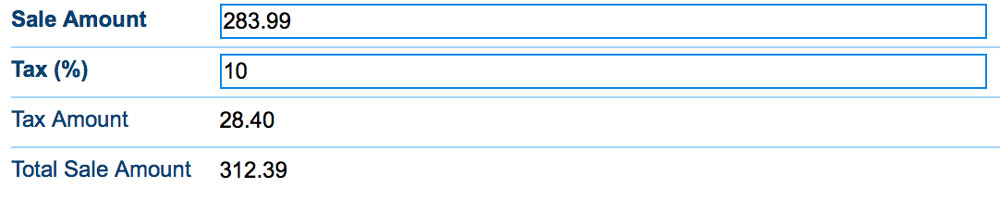#### Instructions

1. Customize the application
2. In the Form section, create a money field to allow the user to enter a pre-tax sale amount eg. 'Sale Amount,' and a numeric field to allow the user to specify a tax percentage eg. 'Tax (%)'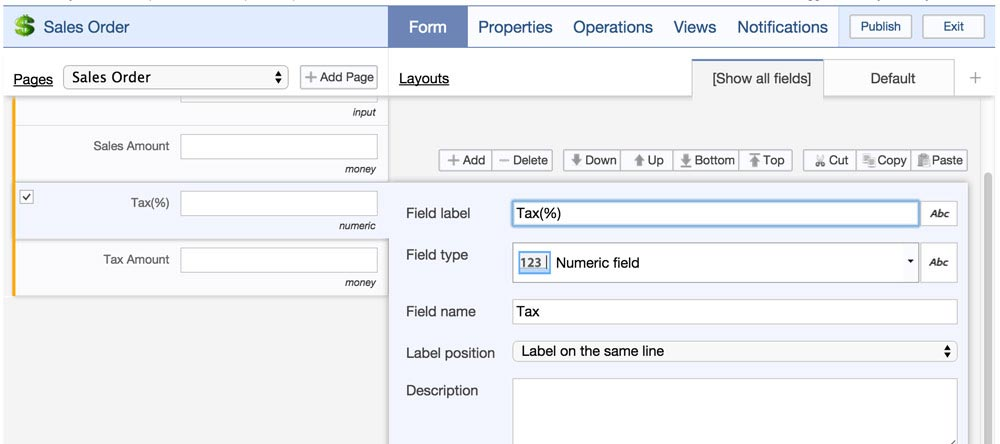3. Note the Field names for the 'Sales Amount' and 'Tax(%)' fields as they will be used for a calculation in the next step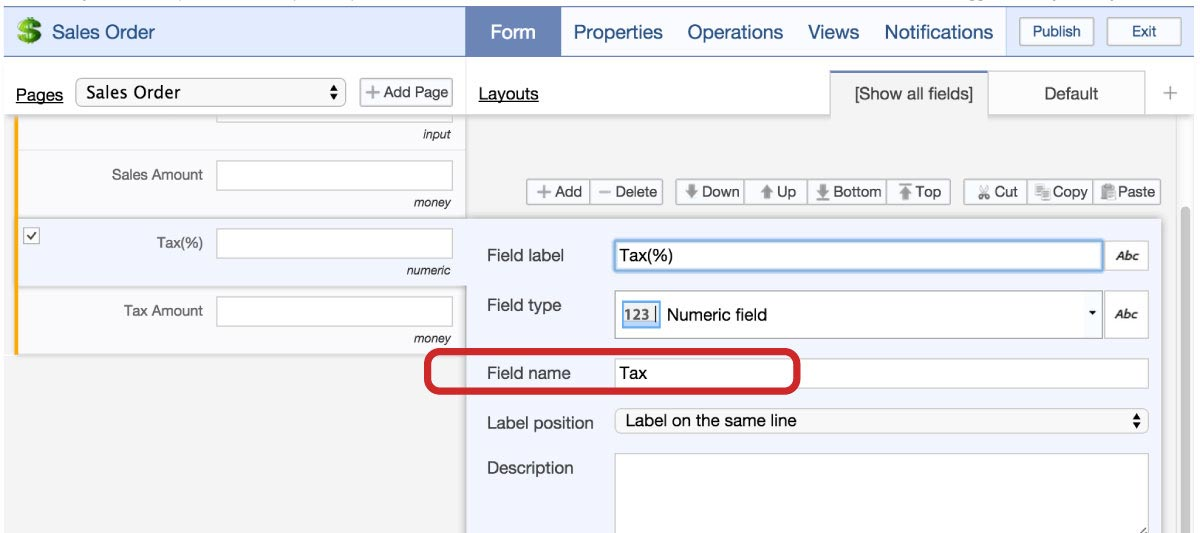4. Create another money field to allow the user to view the tax amount eg. 'Tax Amount'
1. Click on the 'Abc' icon beside the field type, and select 'formula calculation'
2. Enter the formula, using the appropriate field names for the pre-tax sale amount, and the tax percentage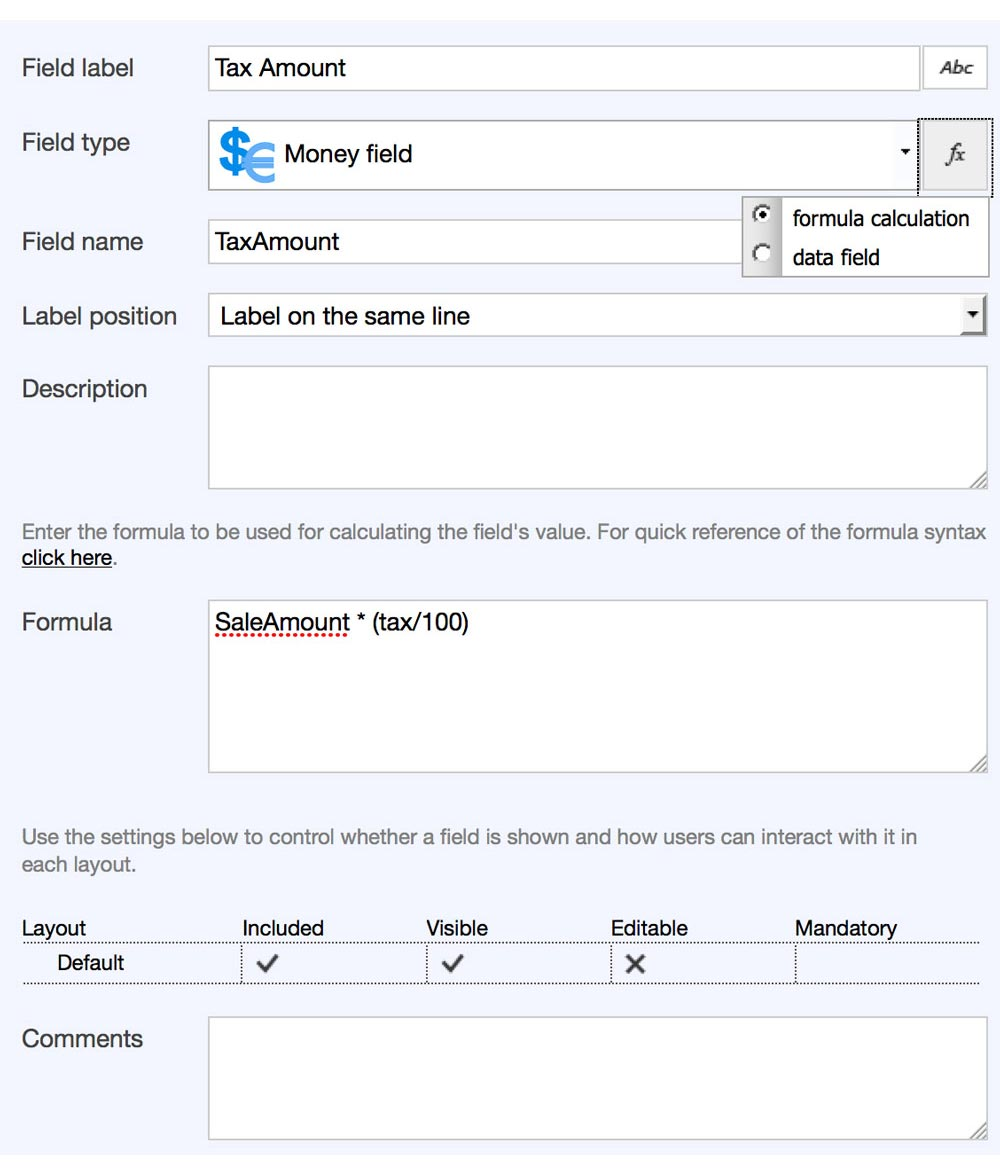5. Create the field to allow the user to view the total sale amount
1. Change the field type to a 'Money Field'
2. Click on the 'Abc' icon beside the field type, and select 'formula calculation'
3. Enter the formula, using the appropriate field names for the pre-tax sale amount, and the tax amount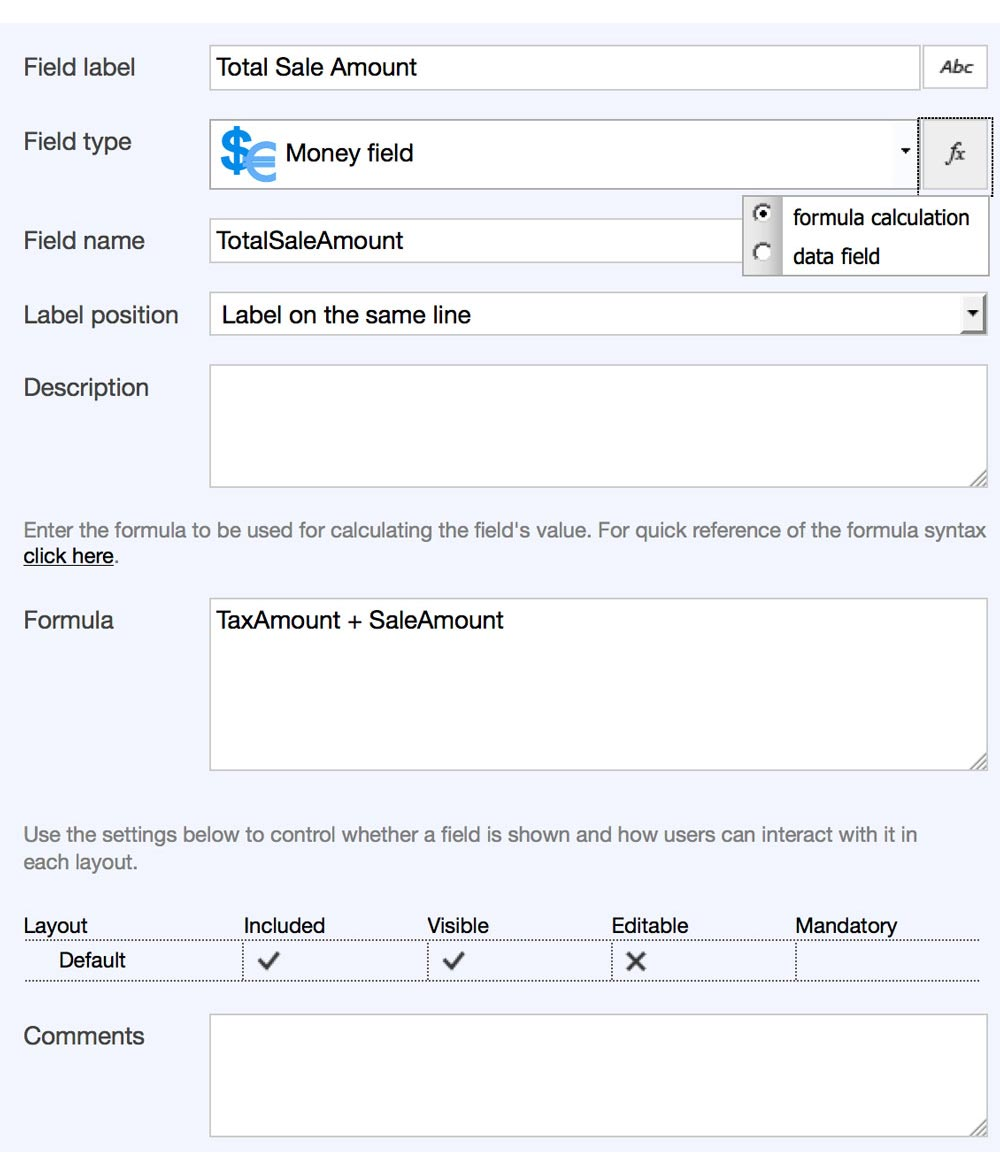6. Save the form, and publish the application